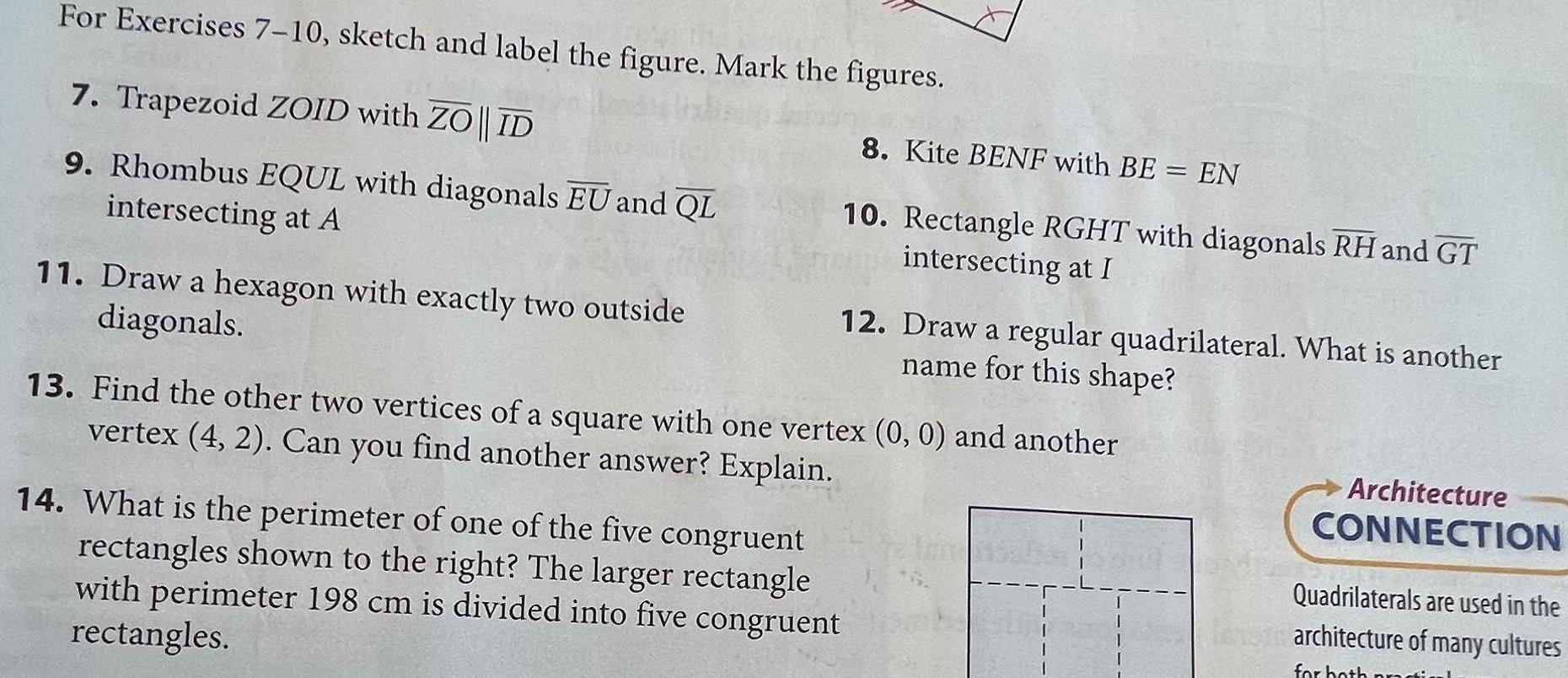Question:

# For Exercises 7 10 sketch and label the figure Mark the

Last updated: 9/19/2023For Exercises 7 10 sketch and label the figure Mark the figures 7 Trapezoid ZOID with ZO ID 9 Rhombus EQUL with diagonals EU and QL intersecting at A 11 Draw a hexagon with exactly two outside diagonals 8 Kite BENF with BE EN 10 Rectangle RGHT with diagonals RH and GT intersecting at I 12 Draw a regular quadrilateral What is another name for this shape 13 Find the other two vertices of a square with one vertex 0 0 and another vertex 4 2 Can you find another answer Explain 14 What is the perimeter of one of the five congruent rectangles shown to the right The larger rectangle with perimeter 198 cm is divided into five congruent rectangles Architecture CONNECTION Quadrilaterals are used in the architecture of many cultures for both pr